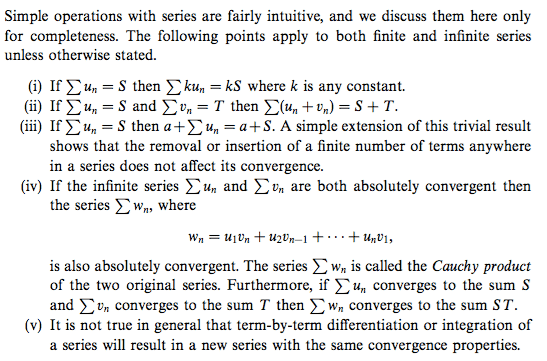# Properties of conditionally convergent series

• I
Do (i), (ii) and (iii) apply to conditionally convergent series as well? I feel like they don't. But the book seems to say that they do because it doesn't "state otherwise".Last edited:

mfb
Mentor
I feel like they don't.
Why do you feel so, do you have a counterexample?
Is there any reordering involved in (i) to (iii)?

Is there any reordering involved in (i) to (iii)?

Yes there is. For (ii), it says ##u_1+u_2+...+v_1+v_2+...=u_1+v_1+u_2+v_2+...##.

There is no reordering in (iii). So we are left to prove that (ii) is true for conditionally convergent series.

If (ii) is true and if (i) is true for ##k=-1##, then (i) is true for all ##k##. Consider ##v_n=\frac{1}{2}u_n##, then using (ii), ##T=S-T##, so ##T=\frac{1}{2}S##. Similarly, we can prove (i) is true for all rational ##k##. Next, we use squeeze theorem to prove that (i) is true for all irrational ##k## too.

Last edited:
mfb
Mentor
Yes there is. For (ii), it says ##u_1+u_2+...+v_1+v_2+...=u_1+v_1+u_2+v_2+...##.
The first thing is not well-defined, and never appears in the problem.
If (ii) is true and if (i) is true for ##k=-1##, then (i) is true for all ##k##. Consider ##v_n=\frac{1}{2}u_n##, then using (ii), ##T=S-T##, so ##T=\frac{1}{2}S##. Similarly, we can prove (i) is true for all rational ##k##. Next, we use squeeze theorem to prove that (i) is true for all irrational ##k## too.
While that is correct, it is way more complicated than necessary. Also, I would think most proofs of the squeeze theorem use one of those properties in some way, making the argument circular.

The first thing is not well-defined, and never appears in the problem.

Is ##(u_1+u_2+...)+(v_1+v_2+...)=(u_1+v_1)+(u_2+v_2)+...## well defined? It says summing up all the ##u##'s and separately all the ##v##'s and then adding up the two sums is equal to summing up all the ##(u+v)##'s. So there is a rearrangement.

mfb
Mentor
That is better, if the "..." are understood as limits.
There is still no rearrangement of the order of the elements in the series, e. g. u5 is always added after u4.

There is still no rearrangement of the order of the elements in the series, e. g. u5 is always added after u4.

But the order we do the sum is different in the RHS from that in the LHS. Isn't this generally not allowed for conditionally convergent series?

mfb
Mentor
As I said, try to find a counterexample. And when you see that every attempt fails, you'll get some insight why the rule is also true for condionally convergent series, and then you can prove it.

Note: conditional doesn't mean every reordering has a different limit. Only some of them. And we still don't reorder within the series here.

Also, I would think most proofs of the squeeze theorem use one of those properties in some way, making the argument circular.

This (elementary, common) proof of squeeze theorem does not use (i), (ii) or (iii): https://proofwiki.org/wiki/Squeeze_Theorem/Sequences/Real_Numbers

My use of squeeze theorem for proving (i) is also true for irrational ##k##:

Suppose ##k_1<k<k_2##, where ##k_1## and ##k_2## are rational and ##k## is irrational. As before, let ##S=\Sigma u_n##.

Since (i) is true for rational ##k_1##,
##\lim_{k_1\rightarrow k}\Sigma k_1u_n=kS##. (And similarly true for ##k_2##.)

Thus,
##kS=\lim_{k_1\rightarrow k}\Sigma k_1u_n\leq \Sigma ku_n\leq\lim_{k_2\rightarrow k}\Sigma k_2u_n=kS##.

By squeeze theorem (or otherwise),
##\Sigma ku_n=kS##.

mfb
Mentor
Since (i) is true for rational ##k_1##,
##\lim_{k_1\rightarrow k}\Sigma k_1u_n=kS##. (And similarly true for ##k_2##.)
How does this step work? You would have to show that you can take out the factor (which is directly what you want to prove) or that limit and sum commute (which is not trivial, and in general false).

Anyway, you are making this way more complicated by going that route. You can directly prove it via the definition of a limit.

How does this step work? You would have to show that you can take out the factor (which is directly what you want to prove) or that limit and sum commute (which is not trivial, and in general false).

##\lim_{k_1\rightarrow k}\Sigma k_1u_n=\lim_{k_1\rightarrow k}k_1\Sigma u_n##, since (i) is true for rational ##k_1##
##=k\Sigma u_n=kS##

Anyway, you are making this way more complicated by going that route. You can directly prove it via the definition of a limit.

I can't prove it directly.

Since ##\lim_{n\rightarrow\infty}\Sigma_{r=1}^nu_r=S##,

##\forall\epsilon>0, \exists N:\,\forall n>N, |\Sigma_{r=1}^nu_r-S|<\epsilon##

##|k|\,|\Sigma_{r=1}^nu_r-S|<|k|\epsilon##
##|k\,\Sigma_{r=1}^nu_r-kS|<|k|\epsilon##

but we want to show that

##|\Sigma_{r=1}^nku_r-kS|<|k|\epsilon,\,\forall n>N##.

This is true if ##k\,\Sigma_{r=1}^nu_r=\Sigma_{r=1}^nku_r,\,\forall n>N## including ##n=\infty##. But this is precisely what we want to prove.

mfb
Mentor
##\lim_{k_1\rightarrow k}\Sigma k_1u_n=\lim_{k_1\rightarrow k}k_1\Sigma u_n##, since (i) is true for rational ##k_1##
##=k\Sigma u_n=kS##
That part works, but how do you prove it is equal to ##\lim_{n \to \infty} \sum_{i=0}^n k u_i##?

I can't prove it directly.
You can. For a finite sum, ##k \sum u_r = \sum k u_r## is trivial (distributive law), and you don't need it for "n=∞" because that never appears in the proof.

That part works, but how do you prove it is equal to ##\lim_{n \to \infty} \sum_{i=0}^n k u_i##?

##\sum_{i=0}^n k_1u_i\leq\sum_{i=0}^n k u_i\leq\sum_{i=0}^n k_2u_i##

By squeeze theorem,
##\lim_{n \to \infty} \sum_{i=0}^n k u_i=kS##

You can. For a finite sum, ##k \sum u_r = \sum k u_r## is trivial (distributive law), and you don't need it for "n=∞" because that never appears in the proof.

##\forall n>N## means we only need to consider finite values of ##n##?

mfb
Mentor
##\forall n>N## means we only need to consider finite values of ##n##?
n is a natural number. Every natural number is finite.

•Happiness